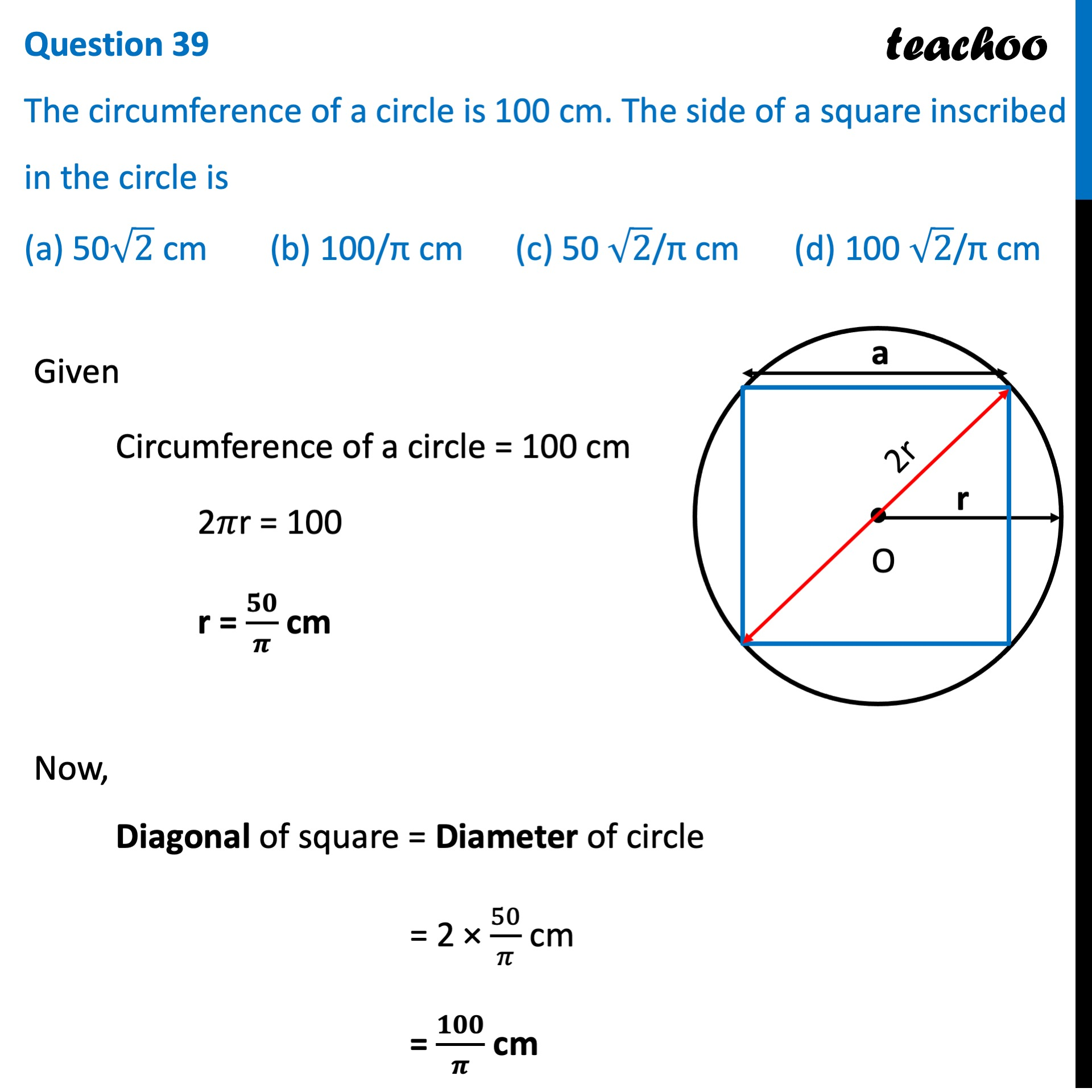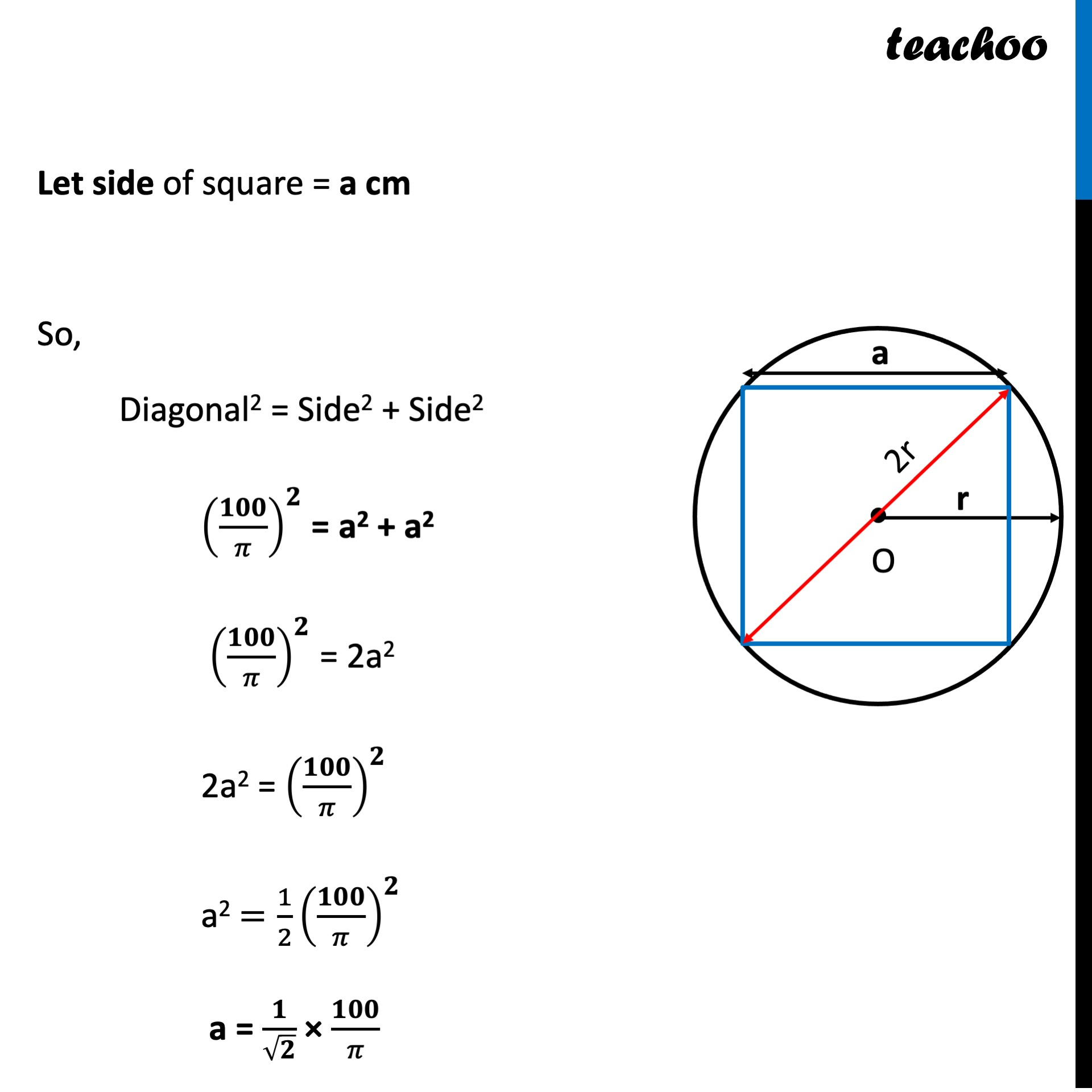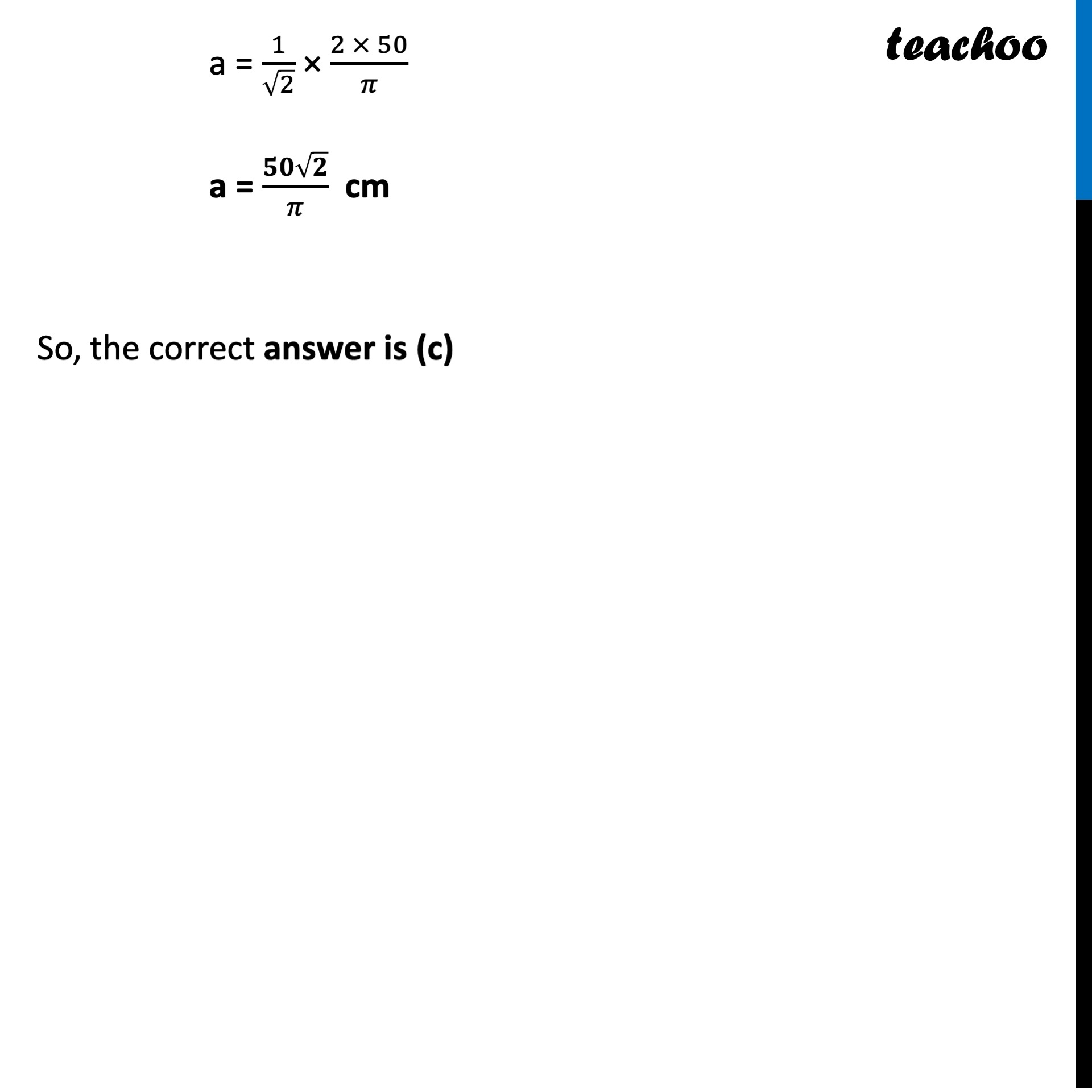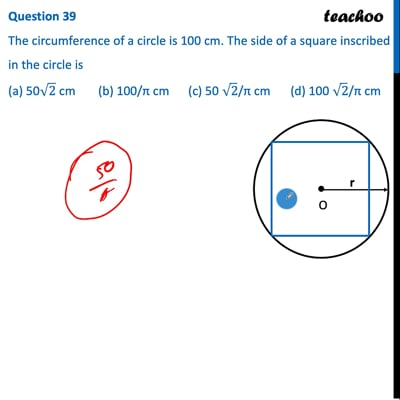CBSE Class 10 Sample Paper for 2022 Boards - Maths Standard [MCQ]

Class 10
Solutions of Sample Papers for Class 10 Boards

## (a) 50√2 cm   (b) 100/π cm   (c) 50 √2/π cm       (d) 100 √2/π cmThis video is only available for Teachoo black users

### Transcript

Question 39 The circumference of a circle is 100 cm. The side of a square inscribed in the circle is (a) 50√2 cm (b) 100/π cm (c) 50 √2/π cm (d) 100 √2/π cm Given Circumference of a circle = 100 cm 2𝜋r = 100 r = 𝟓𝟎/𝝅 cm Now, Diagonal of square = Diameter of circle = 2 × 50/𝜋 cm = 𝟏𝟎𝟎/𝝅 cm Let side of square = a cm So, Diagonal2 = Side2 + Side2 (𝟏𝟎𝟎/𝜋)^𝟐 = a2 + a2 (𝟏𝟎𝟎/𝜋)^𝟐 = 2a2 2a2 = (𝟏𝟎𝟎/𝜋)^𝟐 a2 =1/2 (𝟏𝟎𝟎/𝜋)^𝟐 a = 𝟏/√𝟐 × 𝟏𝟎𝟎/𝜋 a = 1/√2 × (2 × 50)/𝜋 a = (𝟓𝟎√𝟐)/𝜋 cm So, the correct answer is (c)